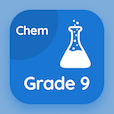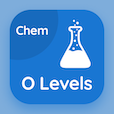SAT Prep Courses

SAT Chemistry MCQs

SAT Chemistry MCQ - Topics

Learn Molecular Formula Multiple Choice Questions (MCQ Quiz), Molecular Formula quiz answers PDF to learn sat chemistry course for sat chemistry online classes. Mole Concept Multiple Choice Questions and Answers (MCQs), Molecular Formula quiz questions for college entrance test. Molecular Formula Book PDF: empirical formula, molar mass test prep for online career assessment.

"Molecular formula of glucose is" MCQ PDF: molecular formula App APK with cho, ch2o, c6h12o6, and cho2 choices for college entrance test. Study molecular formula quiz questions for merit scholarship test and certificate programs for pre employment screening tests.

## MCQ on Molecular Formula Quiz

MCQ: Molecular formula of glucose is

CHO
CH2O
C6H12O6
CHO2

MCQ: C6H6 is formula of benzene

Empirical
Molecular
Simple
Gram

MCQ: The formula which shows the actual number of atoms of each element in a molecule is called

Empirical formula
Molecular formula
Simple formula
Gram formula

MCQ: Molecular formula is related to empirical formula as

n x empirical formula
n x molecular formula
n x atomic formula
n x molar mass

### More Quizzes on SAT Chemistry Book